Related Articles

# Pie Chart in MATLAB

• Last Updated : 07 Apr, 2021

A Pie chart is a circular graph that is divided into sections and each section represents the proportionate part of the whole. In MATLAB we have a function named pie() which allows us to plot a bar graph.

Syntax:

pie(X)

pie(X) draws a pie chart using the data in X. Each slice of the pie chart represents an element in X.

• Where the sum(X) ≤ 1, then the areas of the pie slices directly specify the values in X pie draws only a partial pie if sum(X) < 1.
• Where the sum(X) > 1, then the area of each slice of the pie is determined by pie normalizes the values by X/sum(X)
• Here let X be a categorical data type, the slices correspond to categories. The number of elements in the category divided by the number of elements in X becomes The area of each slice.

Now let’s move to some examples.

Example 1: A simple pie chart:

## MATLAB

 `% data ``b= [20 30 40 30]`` ` `% pie function to draw pie chart``pie(b)`

Output :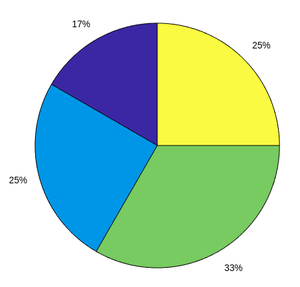fig 1: Pie chart

Example 2: Pie chart with offset:

## MATLAB

 `% data``b= [20 30 40 30]``% offset first and third by using 1 at explode ``explode = [1 0 1 0]`` ` `% pie function to draw pie chart with``% explode 1st and 3rd position data``pie(b,explode)`

Output :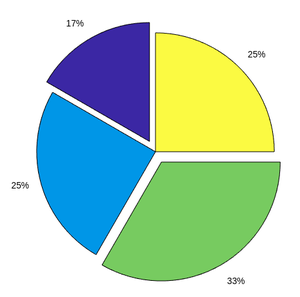fig 2: Pie chart with offset

Example 3: Pie chart with labels:

## MATLAB

 `% data``b= [20 30 40 30]`` ` `% labelling on Pie chart``labels={``'a'``,``'b'``,``'c'``,``'d'``}`` ` `% pie function to draw pie chart with labels``pie(b,labels)`

Output :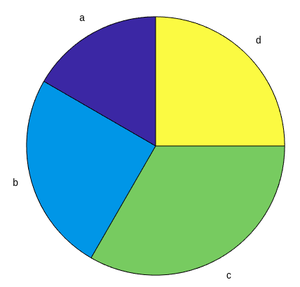fig 3: Pie chart with labels

Example 4: Partial Pie chart:

## MATLAB

 `% create vector whose sum is less than 1``b= [0.2 0.4 0.1]`` ` `% pie function to draw pie chart``pie(b)`

Output :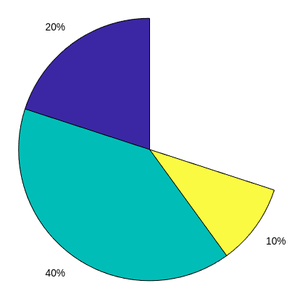fig 4: Partial Pie chart

Example 5: Compare 2 pie charts:

## MATLAB

 `% data``b= [20 30 40 30]``a= [10 40 20 20]`` ` `% labelling on pie chart``labels={``'a'``,``'b'``,``'c'``,``'d'``}`` ` `% to add more than 1 plot in same figure``t = tiledlayout(1,2,``'TileSpacing'``,``'compact'``);`` ` `% create pie charts``ax1 = nexttile;``pie(ax1,a)``title(``'Pie chart 1'``)``ax2 = nexttile;``pie(ax2,b)``title(``'Pie chart 2'``)`` ` `% create legend and put labels as arguments``lgd = legend(labels);``% position of legend there are 4 positions``%'north','south','east','west'``lgd.Layout.Tile = ``'north'``;`

Output :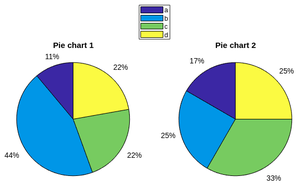fig 5: Comparison of 2 pie charts

My Personal Notes arrow_drop_up